• tarjan求强连通分量
2018-12-03 20:50:00

# 什么是强连通分量？

## 百度百科

有向图强连通分量：在有向图G中，如果两个顶点vi,vj间（vi>vj）有一条从vi到vj的有向路径，同时还有一条从vj到vi的有向路径，则称两个顶点强连通(strongly connected)。如果有向图G的每两个顶点都强连通，称G是一个强连通图。有向图的极大强连通子图，称为强连通分量(strongly connected components)。

# 代码

#include <bits/stdc++.h>
using namespace std;
map<int,int> dfn,low,vis,color;
stack<int> st;
int sum,col;
vector<int> G;
void tarjan(int n)
{
dfn[n]=low[n]=++sum;
vis[n]=1;
st.push(n);
for(int i=0;i<G[n].size();i++)
{
int p=G[n][i];
if(!dfn[p])
{
tarjan(p);
low[n]=min(low[n],low[p]);
}
else if(vis[p])
low[n]=min(low[n],low[p]);
}
if(low[n]==dfn[n])
{
color[n]=++col;
vis[n]=0;
while(st.top()!=n)
{
color[st.top()]=col;
vis[st.top()]=0;
st.pop();
}
st.pop();
}
}
int main()
{
ios::sync_with_stdio(0);
cin.tie(0);
cout.tie(0);
int n,m;
cin>>n>>m;
while(m--)
{
int t1,t2;
cin>>t1>>t2;
G[t1].push_back(t2);
}
for(int i=1;i<=n;i++)
if(!dfn[i])
tarjan(i);
for(int i=1;i<=n;i++)
cout<<color[i]<<" ";
}

转载于:https://www.cnblogs.com/baccano-acmer/p/10060806.html

更多相关内容
• ## Tarjan求强连通分量

千次阅读 2019-04-22 21:53:18
[算法定义] 在有向图中，如果两个顶点至少存在一条路径（可以相互...非连通有向图的极大连通子图，称为强连通分量(strongly connected components)。 在上图中，{1 , 2 , 3 , 4 } , { 5 }　, { 6 } 三个区域...

### [算法定义]

在有向图中，如果两个顶点至少存在一条路径（可以相互通达），则称两个顶点强连通(strongly connected)。

如果有向图G的每两个顶点都强连通，称G是一个强连通图

非强连通有向图的极大强连通子图，称为强连通分量(strongly connected components)。

###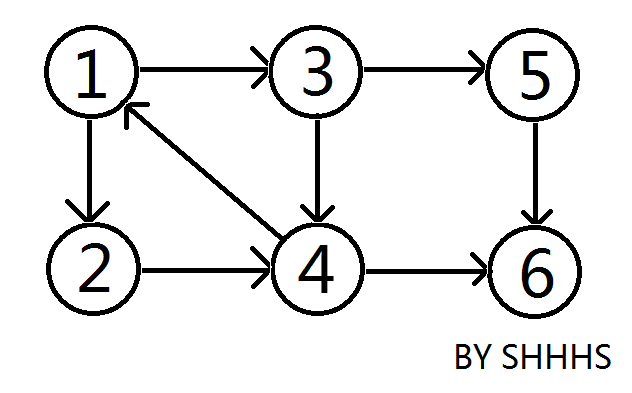在上图中，{1 , 2 , 3 , 4 } , { 5 }　,  { 6 } 三个区域可以相互连通，称为这个图的强连通分量。

Tarjan算法是基于对图深度优先搜索的算法，每个强连通分量为搜索树中的一棵子树。

DFN[ i ] : 在DFS中该节点i被搜索的次序(时间戳)。

LOW[ i ] : 为i或i的子树能够追溯到的最早的栈中节点的次序号。

当DFN[ i ]==LOW[ i ]时，已i为根的搜索子树上所有节点是一个强连通分量。

【心得】：如果有环，dfn是传递自己的下一代，low是继承自己的上一代或自己（上一代无环）

搜索时，把当前搜索树中未处理的节点加入一个堆栈。

回溯时可以判断栈顶到栈中的节点是否为一个强连通分量。

### [算法图解]

从1开始dfs搜索，把遍历到的节点加入栈中。

搜索到i=6时，节点都入栈了，此时就进行回溯。

DFN=LOW，以节点6为根的搜索子树是一个强连通分量（节点6没有子树）。

6出栈。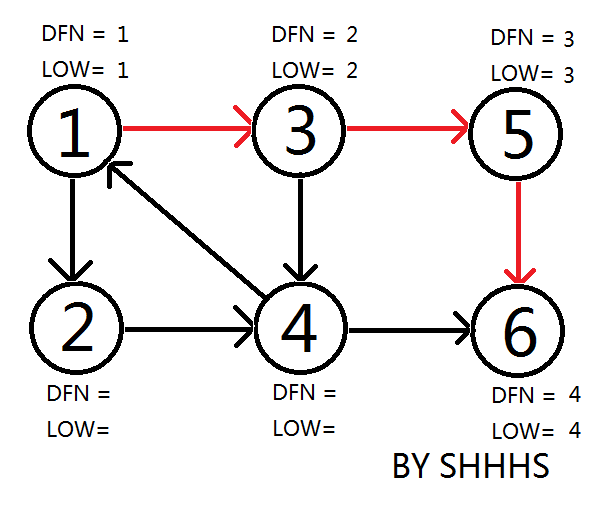依次类推，DFN=LOW，5为强连通分量

并且5的边也都找完了，5出栈。

接下来回溯到3，4入栈。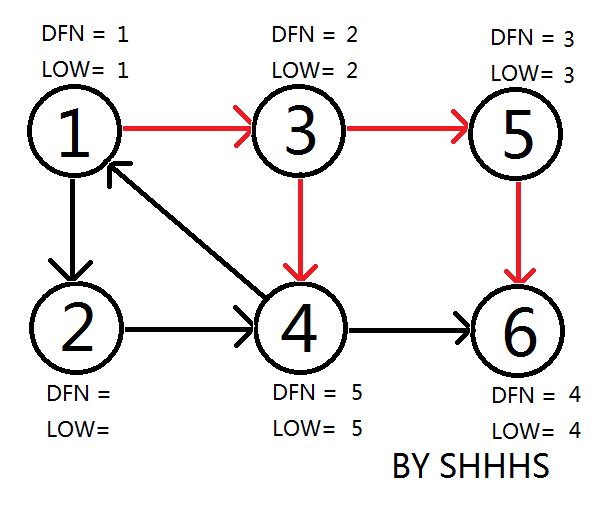然后从4找到1，发现节点1已存在。

将1看做根节点往回搜索子节点，子节点LOW[i]=low[根]=1。

子节点low继承的是根的dfn，根的low就是根的dfn，最小的那个

现在只是将1，3，4看做环，1的边还没有找完。

没有找完自然不会进行根节点1成环的回溯出栈操作。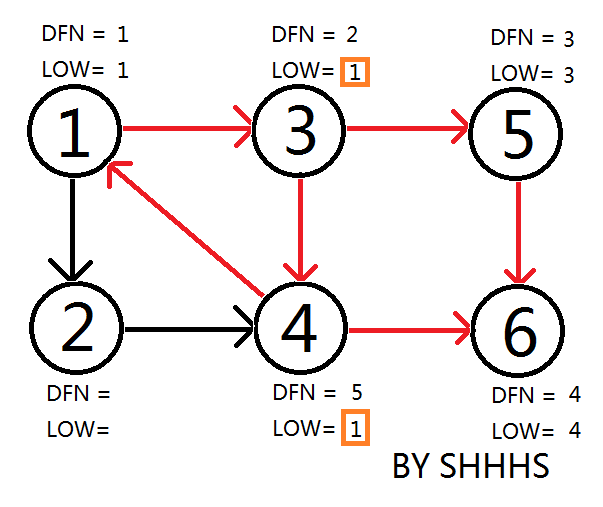继续找1的边，找到2。

再访问4（还在栈中），所以LOW=DFN=5。

那么4的根是2，为什么不继承1的dfn，是为了让缩点与割点代码一致

结果不影响，连通分量还是一起出栈（并染色）

从2返回1后，发现DFN=LOW，把栈中节点全部取出，组成一个强连通分量{1，2，3，4}。

【例】如果2跟1不成环，那么2不会连4，（2将自己抛出）或（2与2的子节点成环整个抛出）

最后执行到1，再进行1的回溯，组成强连通分量{1，3，4}出栈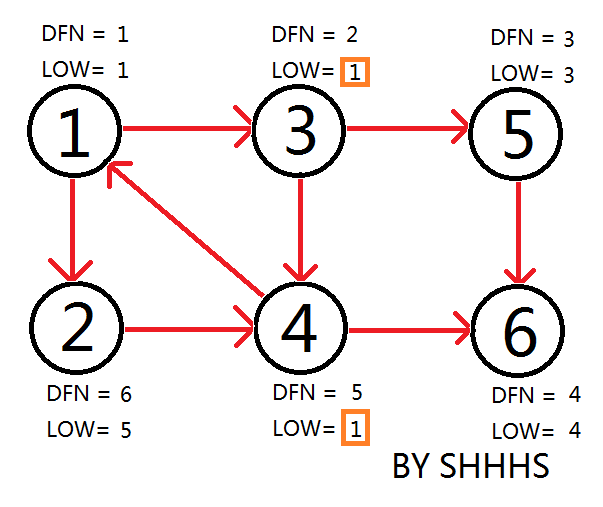自此算法结束，找到{1，2，3，4}，{5}，{6}三个强连通分量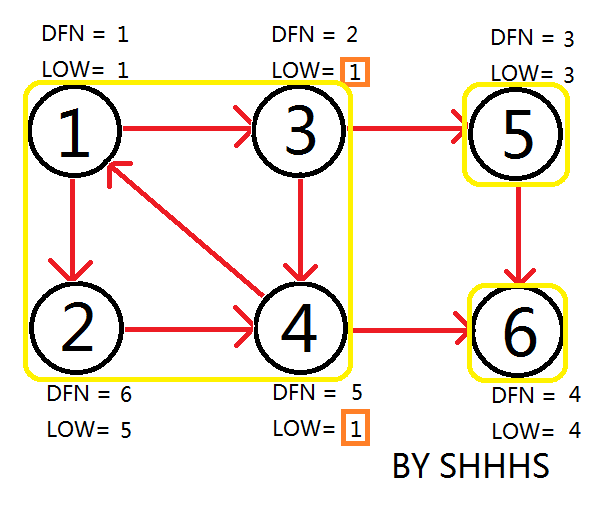### [代码1]不要慌全解系列

#include<iostream>
#include<algorithm>
#include<cstring>
#include<stack>
using namespace std;

struct ss{
int v;
int next;
/*
v指节点v
next永远指u点->上一个点的边序（1，2，3···）
边序 即u到其他点（上一个点）是第几条边（num）
上一条边没有就是-1
*/
}s;

int dfn;
int low;
int vis;//相当于栈
int color;//染色
int n,m;
int cnt;
int num;
stack<int >st;

void init()
{
memset(vis,0,sizeof(vis));
memset(dfn,0,sizeof(dfn));
memset(low,0,sizeof(low));
//memset(color,0,sizeof(color));
num=0;
cnt=0;
}
{
s[num].v = v;
/*
将v存进去
将u->上一个点的边序挂上next
num一直在++(总边数不会错)

如果双向再存u挂v边序
eg[num].v = u;
*/
}
void Tarjan(int u)
{
st.push(u);
dfn[u] = low[u] = ++cnt;//搜索次序号
vis[u]=1;//节点x在栈中
for(int i = head[u]; i != -1; i = s[i].next)
{
//通过对u点上一个边序的挂钩
//构造对连接u点的所有边数遍历查找对应v点
int v = s[i].v;
if(!dfn[v])//不曾访问过
{
Tarjan(v);//找v点
low[u] = min(low[u],low[v]);
/*
根节点的dfn值是区分不同环的值
low是为了让这个环都等dfn[根]
low[根]可能不等dfn[根]
根的low继承根的根的dfn
1.如果v是根节点
不论只有v一个点还是有一个环
low[v]确实永远比low[u]大(u比v先入)
v的环low值=dfn[v]都比low[u]的大
v不对u产生影响
2.
如果v点与u点成环
那么顺着v点或v连着的点找下去
总有一个能连到根节点
low值回溯的时候继承根的dfn值
根的dfn是这个环里面最小的
low[v]等于dfn[根]
v对u产生影响->low[u]=low[v]
*/
}
else if(vis[v])//访问过但还在栈中
/*
因为根u点还没有将边都找完
出栈的点都是根节点边已经找完的点或者环
已经没有与剩下的点有任何关系才能出
*/
low[u] = min(low[u],dfn[v]);
/*
这相当于根节点有两个分叉口a,b
并且a找到已经在栈中的b

那么这一步其实也可以写成
low[u] = min(low[u],low[v]);
反正连到一个环了

目的是为了让缩点与割点的代码一致
区分相连的环的根有不同的dfn
无向图找割点用的

但是缩点是将一起出栈的点缩成一个点（染成一个色）
对于缩点结果都无影响
*/
}

if(dfn[u]==low[u])//找一遍再是强连通分量
{
int now;
do{	//取出包括u的环
now=st.top();
color[now]=u; //染色
vis[now]=0;
st.pop();
}while(now!=u);
}
return;
}
void out()
{
for(int i=1;i<=n;i++)
printf("%d ",i);
printf("\n");
for(int i=1;i<=n;i++)
printf("%d ",color[i]);
printf("\n");
}

int main()
{
while(~scanf("%d%d",&n,&m) && (m+n))
{
init();
int u,v;
while(m--)
{
scanf("%d%d",&u,&v);
}
//为了防止一个图里有不相连的两个或多个树
for(int i=1;i<=n;i++)
if(!dfn[i])
Tarjan(i);
out();
}
return 0;
} 

### [代码2]

若只是缩点，求强连通分量染色，不与割点代码一致，就不需要用栈

#include<iostream>
#include<algorithm>
#include<cstring>
#include<stack>
#include<vector>
using namespace std;

int dfn;
int low;//就相当于颜色，一个环一个low=dfn[根]
int vis;
int n,m;
int cnt;
stack<int >st;
vector<int >vc;

void init()
{
memset(vis,0,sizeof(vis));
memset(dfn,0,sizeof(dfn));
memset(low,0,sizeof(low));
cnt=0;
}

void Tarjan(int u)
{
st.push(u);
dfn[u] = low[u] = ++cnt;
vis[u]=1;
for(int i = 0; i < vc[u].size(); i++)
{
int v = vc[u][i];
if(!dfn[v])
{
Tarjan(v);
low[u] = min(low[u],low[v]);
}
else
low[u] = min(low[u],low[v]);
}
return;
}
void out()
{
for(int i=1;i<=n;i++)
printf("%d ",i);
printf("\n");
for(int i=1;i<=n;i++)
printf("%d ",low[i]);
printf("\n");
}

int main()
{
while(~scanf("%d%d",&n,&m) && (m+n))
{
init();
int u,v;
while(m--)
{
scanf("%d%d",&u,&v);
vc[u].push_back(v);
}
for(int i=1;i<=n;i++)
if(!dfn[i])
Tarjan(i);
out();
}
return 0;
} 

展开全文• tarjan算法的实现方式 作为一个只进行一次dfs就能找到环并且成功缩点的算法来说，tarjan的dfs还是比较强大的，那么它又具体是怎么实现的呢？ 首先，我们需要明白一个时间戳的概念。命名一个记录时间点的变量idx，...

## tarjan算法的实现方式

作为一个只进行一次dfs就能找到环并且成功缩点的算法来说，tarjan的dfs还是比较强大的，那么它又具体是怎么实现的呢？       首先，我们需要明白一个时间戳的概念。命名一个记录时间点的变量idx，每dfs找到一个点，就将该点的时间戳赋值为时间节点idx，然后用idx++来模拟“时间流逝”，这样就可以记录下每一个节点被遍历到的次序了。

既然时间记录已经完成了，那么这个时间戳又有什么用呢？使用两个数组dfn和low。dfn[u]表示dfs时达到顶点u的次序号（时间戳），low[u]表示以u为根节点的dfs树中次序号最小的顶点的次序号（也就是说low中储存的是当前这个节点所能到达的时间戳最小的点）。除了两个int数组外，还有两个布尔数组instack和vis，前者用来存储该节点是否还在栈中，而后者用来存储这个节点是否有没有访问过（在后面的学习中清务必明确二者的区别）。

在经过一个点low值的一系列更新后，如果这个点找不到dfn比它自己还小的点的时候，也就是当dfn[u]=low[u]时，以u为根的搜索子树上所有节点是一个强连通分量。 先将顶点u入栈，dfn[u]=low[u]=++idx,扫描u能到达的顶点v，如果v没有被访问过，则dfs(v)，low[u]=min(low[u],low[v])，如果v在栈里，low[u]=min(low[u],dfn[v])，扫描完v以后，如果dfn[u]=low[u]，则将u及其以上顶点出栈。（具体的实现过程可以参考一下five20大佬的博客，那里面的几张图对帮助理解很有用处，实在搞不明白的话可以拿起笔和纸照着图画一遍）

最后代码实现的话可以参考一下下面的代码和注释（谢谢five20 qwq）

#include <cstdio>
#include <stack>
#include <cstring>
#include <iostream>
using namespace std;
int n,m,idx=0,k=1,Bcnt=0;
int ins={0};
int dfn={0},low={0};
int Belong;
stack <int> s;
struct edge
{
int v,next;
}e;
int min(int a,int b)
{
return a<b?a:b;
}
{
e[k].v=v;
}
{
int a,b;
scanf("%d%d",&n,&m);
for(int i=1;i<=m;i++)
{
scanf("%d%d",&a,&b);
}
}
void tarjan(int u)
{
int v;
dfn[u]=low[u]=++idx;//每次dfs，u的次序号增加1
s.push(u);//将u入栈
ins[u]=1;//标记u在栈内
{
v=e[i].v;
if(!dfn[v])//如果v没被处理过
{
tarjan(v);//dfs(v)
low[u]=min(low[u],low[v]);//u点能到达的最小次序号是它自己能到达点的最小次序号和连接点v能到达点的最小次序号中较小的
}
else if(ins[v])low[u]=min(low[u],dfn[v]);//如果v在栈内，u点能到达的最小次序号是它自己能到达点的最小次序号和v的次序号中较小的
}
if(dfn[u]==low[u])
{
Bcnt++;
do
{
v=s.top();
s.pop();
ins[v]=0;
Belong[v]=Bcnt;
}while(u != v);
}
}
void work()
{
for(int i=1;i<=n;i++)if(!dfn[i])tarjan(i);
printf("\n");
for(int i = 1;i <= 6;i++)printf("%d %d\n",dfn[i],low[i]);
printf("共有%d强连通分量，它们是:\n",Bcnt);
for(int i=1;i<=Bcnt;i++)
{
printf("第%d个:",i);
for(int j=1;j<=n;j++)
{
if(Belong[j]==i)printf("%d ",j);
}
printf("\n");
}
}
int main()
{
work();
return 0;

展开全文•c++
• 图论- 图的连通性- Tarjan 求强连通分量.rar
• int dfn[maxn], low[maxn], scc[maxn], tot, scc_cnt; stack<int> st; void tarjan(int x) { dfn[x] = low[x] = ++tot; st.push(x); for (auto v:G[x]) { if (!dfn[v]) { ta...
int dfn[maxn], low[maxn], scc[maxn], tot, scc_cnt;
stack<int> st;

void tarjan(int x) {
dfn[x] = low[x] = ++tot;
st.push(x);
for (auto v:G[x]) {
if (!dfn[v]) {
tarjan(v);
low[x] = min(low[x], low[v]);
} else if (!scc[v]) {
low[x] = min(low[x], dfn[v]);
}
}
if (dfn[x] == low[x]) {
++scc_cnt;
int v;
while (v != x) {
v = st.top();
st.pop();
scc[v] = scc_cnt;
}
}
}

void findScc() {
tot = scc_cnt = 0;
memset(dfn, 0, sizeof(dfn));
memset(scc, 0, sizeof(scc));
for (int i = 1; i <= n; ++i) {
if (!dfn[i]) {
tarjan(i);
}
}
}

展开全文• Tarjan 算法求强连通分量Tarjan 算法中为每个结点 u 维护了以下几个变量： dfn[n] ：深度优先搜索遍历时结点 u 被搜索的次序。 low[u] ：设以 u 为根的子树为 Subtree(u) 。low[u] 定义为以下结点的 dfn 的...算法 数据结构
• 网上看了几篇博客，还有OI Wiki，觉得整合度不够，于是特意写了篇博客。 参考资料： 全网最!详!细!Tarjan算法讲解。...强连通分量（Strongly Connected Components，简称SCC）的定义是：极大的连通子图。 举一算法 c++ 数据结构
• 首先介绍一下什么是（有向图）强连通分量—— 在有向图G中，如果两个顶点间至少存在一条路径，称两个顶点连通。如果有向图G的每两个顶点都连通，则称G是一个连通图。非连通图有向图的极大连通子图，成为...
• 一. 什么是强连通分量强连通分量：在有向图G中，如果两个顶点u,v间（u->...在连图图的基础上加入一些点和路径，使得当前的图不在连通，称原来的连通的部分为强连通分量。 二. 连通分算法 c++
• int times , tot; int n; int dfn , low, vis, stack, color, type = 0; int in,out;...void tarjan(int u) { dfn[u] = low[u] = ++ times; stack[++ tot] = u; vis[...
• 强连通分量，是一个有向图的最大连通子图（看起来好像没有什么解释效果…），好吧，强连通分量就是在一个有向图中，从任意一个点出发，最多可以走过的所有的点构成的一个点集，将其称之为连通。强连通分量就是...计算机科学 算法
•算法 c++
• 实现用于查找有向图的强连通分量Tarjan 算法。 在强连通分量 (SCC) 中，每个节点到每个其他节点都有一条路径。 SCC 是不相交的。 入度或出度为零或属于无环图的节点自己形成 SCC。 接受邻接矩阵作为输入。 为了...matlab
• 1.先用Tarjan找到所有强连通分量。然后统计强连通分量的数目、入度为0的强连通分量的数目、出度为0的强连通分量的数目。 2.询问1：入度为0的强连通分量的数目，但需要注意只有一个环的情况。 3.询问2：入度为0和...
• tarjan陪伴联通分量 生成树完成后思路才闪光 欧拉跑过的七桥古塘 让你心驰神往”----《膜你抄》 自从听完这首歌，我就对tarjan开始...
• 强连通分量：有向图的极大连通子图称为强连通分量，注意是极大而非最大，这包含了两个意思：1.有向图的强连通分量可以有多个；2.如果选取了某个子图G2且G2连通，但在图G中还存在一个点v，使得v和G2中任意一个点...
• 1.Tarjan算法求强连通分量 2. Tarjan算法割点 3. Tarjan算法点双连通分量 4. Tarjan算法割边 5.Tarjan算法边双连通分量 1.Tarjan算法求强连通分量 了解一下 强连通分量 对于一个有向图的DFS的搜索树...边双连通分量
• 题意：n个点m条单向边，问任意两个点是否连通。 总结：参考大神博客码的，有些地方还是不太明白。 而且这题还可以双向dfs做，有时间再做一下。 // HDU-1269 #include<bits/stdc++.h> using namespace...
• ）图作为输入，并以拓扑顺序返回其强连通分量作为输出 循环依赖 在各种情况下，依赖关系可能是循环的，并且必须同时执行一组相互依赖的操作。同时执行成本高昂的情况并不少见。使用 Tarjan 算法，可以确定执行相互...
• Tarjan算法求强连通分量强连通分量就是有向图(可以是子图)中的任意两点都能互相到达，所以我们可以用Tarjan算法去出所有的强连通分量，相当于缩点，然后把这些缩点连接起来，就是DAG(有向无环图)，DAG一定有一个...
• tarjan求强连通分量模板。 代码 #include <bits/stdc++.h> using namespace std; const int N = 10000 + 5; vector<int> edge[N]; int dfn[N], low[N], ct, cnt; bool instack[N]; stack<int> st...
• 使用Tarjan算法进行快速计算强连通分量，C++语言实现。...